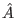Next: Proof that Operations are Up: Grover's Algorithm Previous: Operator to Rotate Phase   Contents

The matrix representation of the inversion about average operatoris defined: Aij = 2/N if ij and Aii = - 1 + 2/N. Note that A = - I + 2P where I is the identity matrix, and P is the matrix with each element is equal to 1/N. Observe that P has the following two properties, first P2 = P, and second Pv, for any vector v, results in a vector v' with each element being the arithmetic average of the the elements of v. [Grover96]